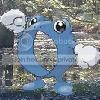-=+=- -=+=- -=+=- -=+=- -=+=- -=+=- -=+=- -=+=- -=+=- -=+=- -=+=- -=+=- -=+=- -=+=- -=+=- -=+=- -=+=- -=+=- -=+=- -=+=- -=+=- -=+=- -=+=- -=+=- -=+=- -=+=- -=+=- -=+=- -=+=- -=+=- (c) WidthPadding Industries 1987 0|604|0 -=+=- -=+=- -=+=- -=+=- -=+=- -=+=- -=+=- -=+=- -=+=- -=+=- -=+=- -=+=- -=+=- -=+=- -=+=- -=+=- -=+=- -=+=- -=+=- -=+=- -=+=- -=+=- -=+=- -=+=- -=+=- -=+=- -=+=- -=+=- -=+=- -=+=-

irondirgible# Zero Punctuation?

13th September 2008

He's funny as ever, but I miss the vaguely-associated songs at the beginning and ending of each video (e.g., [i]In the Navy[/i] for Bioshock).

'Might have been some sort of syndication problem, though.

irondirgible# Text in C++

12th August 2007

I recently started programming in C++ from a book and an online SDL tutorial. As many of you may know, basic SDL does not have font- or text-related functions. I spent several days looking for solutions, trying FreeType2 and SDL_ttf. Even with the proper h*snip*

irondirgible# Play Station!

7th June 2007

How are you supposed to hold the controller? If it was held as one would expect (cord coming out of the top, d-pad in left hand) it appears that the controller would be in a u-shape. Wierd.

Can anyone see the fifth and sixth face buttons? I'm intereste*snip*

irondirgible# May 2007 - "Other Skills" month

16th May 2007

@garand: I did those for 3 years (4th through 6th grade). I never did much of anything for my team because I was the youngest (and my brother was also on it).

I don't have any real 'hobbies' outside of programming (gave up piano in the 4th grade, regre*snip*

irondirgible# #099 - The Balloon Challenge

15th May 2007

[url]https://www.fileden.com/files/2006/8/9/161039/pokey.PNG[/url]
[url]https://www.fileden.com/files/2006/8/9/161039/pokey.bb[/url]

Found out about the contest yesterday. I wrote much of it during school. It's called "Pokey" (for lack of a better name*snip*

irondirgible# Age

11th April 2007

Fifteen (July 7). My Dad's birthday is also April 8th (He turned 50). He is a coder as well as me, although he was a music major in college.

More - Older Posts